Asymptotic equality

(diff) ← Older revision | Latest revision (diff) | Newer revision → (diff)

Two functions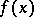andare called asymptotically equal asif in some neighbourhood of the point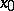(except possibly atitself)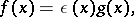where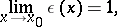i.e.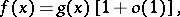as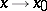(is a finite or an infinite point of the set on which the functions under consideration are defined). If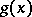does not vanish in some neighbourhood of, this condition is equivalent to the requirement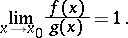In other words, asymptotic equality of two functionsandasmeans, in this case, that the relative error of the approximate equality ofand, i.e. the magnitude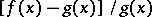,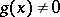, is infinitely small as. Asymptotic equality of functions is meaningful for infinitely-small and infinitely-large functions. Asymptotic equality of two functionsandis denoted by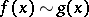as, and is reflexive, symmetric and transitive. Accordingly, the set of infinitely-small (infinitely-large) functions asis decomposed into equivalence classes of such functions. An example of asymptotically-equal functions (which are also called equivalent functions) asare the functions,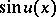,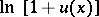,, where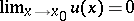.

If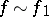and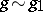as, then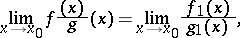where the existence of any one of the limits follows from the existence of the other one. See also Asymptotic expansion of a function; Asymptotic formula.

One also says thatandare of the same order of magnitude atinstead of asymptotically equal.